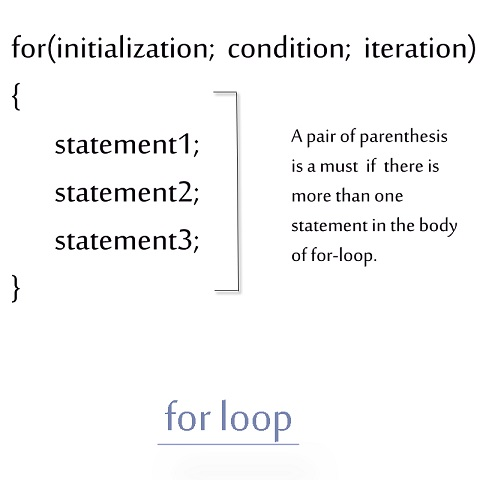< Prev
Next >

# C++ For Loop

C++ language provides us a powerful loop through which we could execute statements in a loop(again and again), until the point when the boolean condition of the loop returns true. This loop is known as for loop and it has three segments -

• The first segment - Initialization i.e. initializing the loop counter to a specific value.

• The second segment - Condition i.e. a boolean condition testing the value of counter. The statements in for loop are executed only if this condition is true.

• The third segment - Incrementation i.e. incrementing or iterating the value of counter each time after the statements in for loop are executed.• ## for loop example

• ``````// C++ for loop example

#include<iostream>

using namespace std;
int main() // main method starts
{

for(int counter = 0; counter<3; counter++)
{
cout<<"counter = " <<counter <<"\n";
}

return 0;
}// main method ends and program ends too
``````

## Output

``````counter = 0
counter = 1
counter = 2``````

• ## For loop with a missing initiailization part or boolean condition in its declaration.

• There can even be a for loop with missing initialization part or boolean condition part in its declaration. But, in order to make this for loop work properly, we will have to use break statement at the right time. Let's see an example.

``````// C++ for loop example

#include<stdio.h>

using namespace std;
int main() // main method starts
{

for(int counter = 0;; )
{
if(counter<5)
{
cout<<"counter = "<<counter <<"\n";
counter++;
}
else
break;	// In this case, break statement makes the for loop work

return 0;
}

} // main method ends and program ends too
``````

## Output

``````counter = 0
counter = 1
counter = 2
counter = 3
counter = 4``````

Note : If you remove the break statement from our last program, the program gets hanged. Hence, the use of break statement is utmost important when we have left off the initialization part or boolean condition part of for loop.

• ## For loop does not need to have an iteration part in its declaration

The third segment of the for loop declaration i.e. iteration of counter can be intentionally left blank from its declaration and can be specified within the body of for loop. In other words, the counter of for loop can also be incremented in the body of for loop.

``````// C++ for loop example

#include<iostream>

using namespace std;
int main() //main method starts
{

for(int counter = 0; counter<3; ) // counter iteration part is intentionally left
{
cout<<"counter = " <<counter <<"\n";
counter++;		  // counter is incremented/iterated in the body of for-loop
}

return 0;
}// main method ends and program ends too
``````

## Output

``````counter = 0
counter = 1
counter = 2``````

< Prev
Next >
< Nested If Else Statement
Do-While Loop>